×#### Thank you for registering.

One of our academic counsellors will contact you within 1 working day.

Click to Chat

1800-1023-196

+91-120-4616500

CART 0

• 0

MY CART (5)

Use Coupon: CART20 and get 20% off on all online Study Material

ITEM
DETAILS
MRP
DISCOUNT
FINAL PRICE
Total Price: Rs.

There are no items in this cart.
Continue Shopping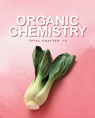```Solved Examples on Isomerism

Question 1.  How many chain isomers does butylene have?

Solution:  Butylene have two chain isomers,

1. n-Butane : CH3CH2CH=CH2

2.  Isobutylene: CH3C(CH3)=CH2

Question 2. How many chain isomers does propyl benzene have?

Solution: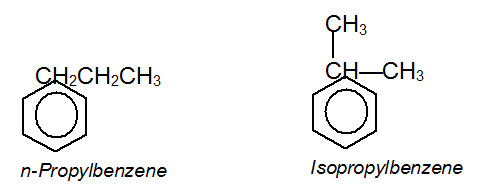Question 3. Give the possible chain isomers for ethyl benzene.

Solution: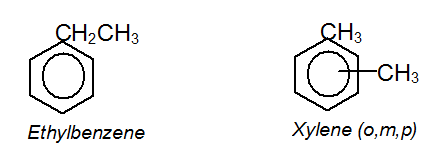Question 4. State whether the following compound is optically active or inactive.The molecule contains one chiral carbon and so must be optically active. Isotopes are regarded as different atom

Question 5. The specific rotation of R— (—) –2– bromooctane is –36°. What is percentage composition of a mixture of enantiomers of 2-bromooctane where rotation is +18°?

Solution.

Let X = mole fraction of R,

=> (1–X) = mole fraction S.

X(–36°) + (1–X) 36° = 18°

or, X = 1/4

The mixture has 25% R and 75%, it is 50% racemic and 50% S.

Question 6 : C4H8 can have so many isomers. Write their structures. What are different tests to make distinction between them

Solution:

C4H8 can have following structures:

(a) CH3—CH2—Ch=CH2 1-butene

(b) CH3—CH=CH—CH3 2-butene, (cis and trans)

(c)  CH3—C(CH3)=CH2 2-methyl-1-propene

(d) Cyclobutane

(e) methyl cyclopropane

Distinctions:

i)  Ozonolysis of (a) forms CH3CH2CHO and HCHO or (b) forms CH3CHO of (c) forms (CH3)2C=O and HCHO

ii)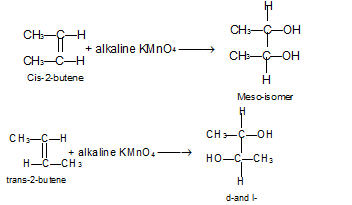Question 7 : Arrange the following species in increasing order of dipole moment.

Cis – 2, 3 –dichloro-2-butene
Cis – 1,2 –dichloroethene
Cis-1,2-dibromo-1,2-dichloroethene
Trans -1,2 -dichlorethene?

Solution: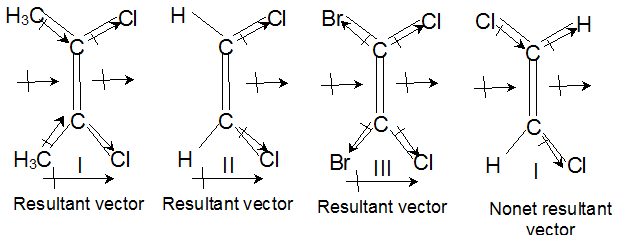In I, there is addition of vector

In II, there will neither be addition nor subtraction of vector

In III, there is subtraction of vector

In IV, the vectors almost cancel each other. So the increasing order of dipole moment is IV < III < II < I.

Question 8: Write all possible structural isomers of the compound with molecular formula C5H12O.

Solution: Total number of structural isomers = 7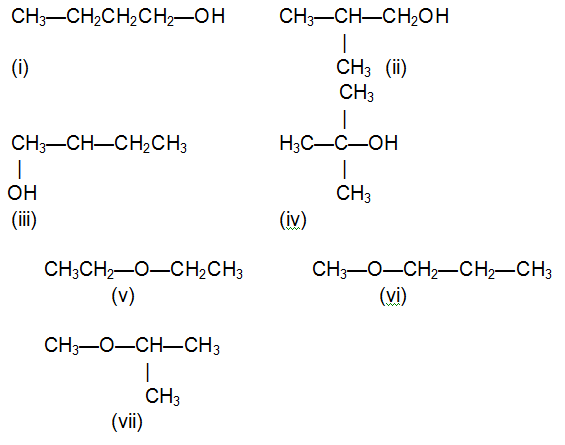Question 9: Write the following Alkenes in increasing order of their stability  with explanation

R2C=CR2, R2C=CHR, R­2C=CH2, RCH=CH2, CH2=CH2

Solution: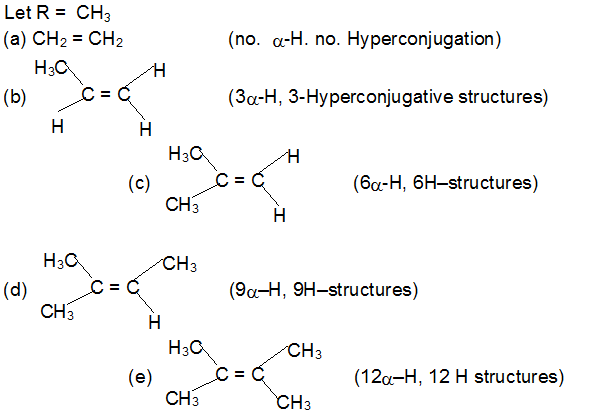From (a)  to (e) number of hyperconjugative structures increases, hence stability of alkene also increases, hence the correct order is a <  b < c < d < e.

Question 10: The compound having molecular formula C4H10O can show

Metamerism

Functional isomerism

Positional isomerism

All

Solution:  Alcohols show position isomerism; Ethers show metamerism; Alcohol and ethers shows functional isomerism. Hence, the correct option is 4.

Click here to refer  Revision notes on Organic Chemistry, IIT JEE Organic Chemistry Syllabus and Chemistry books
```### Course Features

• 731 Video Lectures
• Revision Notes
• Previous Year Papers
• Mind Map
• Study Planner
• NCERT Solutions
• Discussion Forum
• Test paper with Video Solution